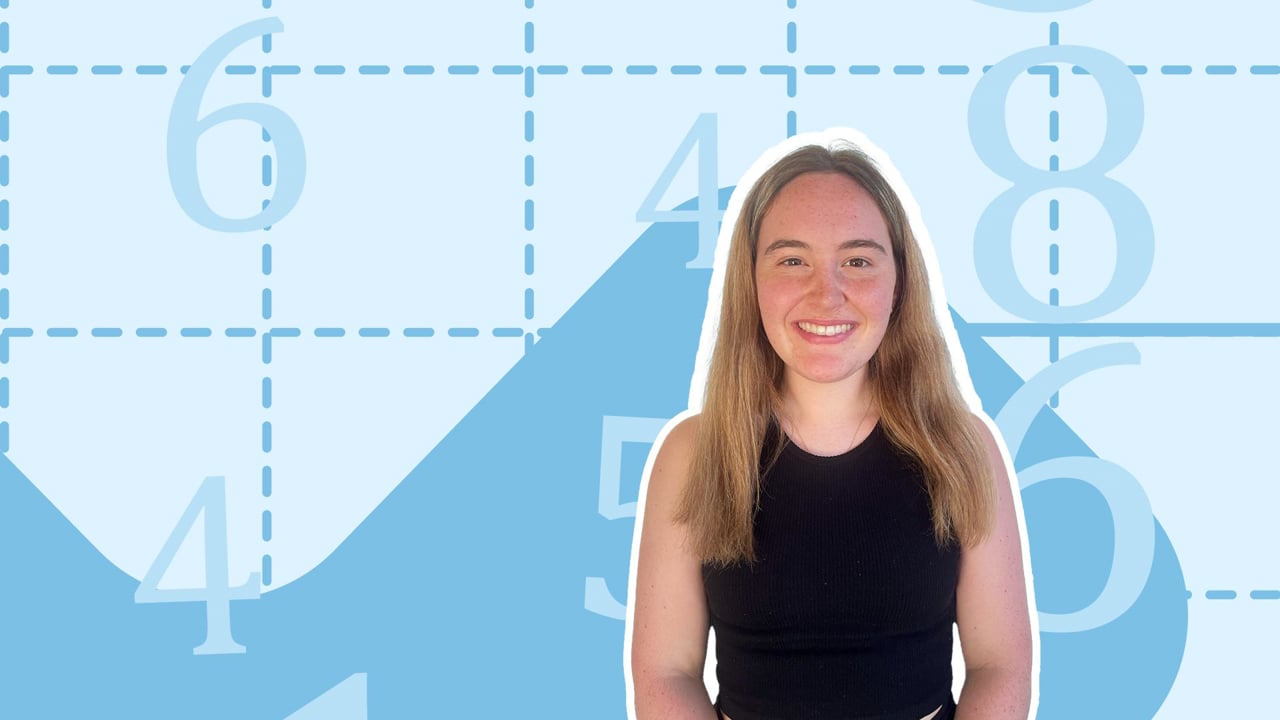Chapter OverviewMaths

Number and place value

Multiplication and division

Fractions

Measurement

Geometry - properties of shapes

Geometry - position and direction

Statistics

Maths

# Counting in powers of 100%

Summary

# Counting in powers of 10

## ​​In a nutshell

Counting in powers of $10$ involves multiplying a number by $10$ over and over, noting down the result each time.

## How to count in powers of 10

When counting in powers of ten, a number is multiplied by $10$ repeatedly. How you do this depends on whether your number is a decimal or a whole number.

### Count in powers of $10$​ for decimal numbers.

#### Procedure

 1 Choose the first number moving along the place value columns that is not zero. 2 Move that digit (and every digit to the right of it) one place value to the left. 3 Continue until every digit has been moved after the decimal point.

Note: Zeros to the left of the first digit after the zero do not need to written down under columns greater than the ones.

##### Example 1

Count in powers of $10$ from $0.072$ to $72$.

The first number after the decimal place that is not zero is seven.

$\begin{array}{ccccccc} \text{Hundreds} & \text{Tens} & \text{Ones} &.&\text{Tenths} &\text{Hundredths}&\text{Thousandths} \\ & & 0& . & 0 & \boxed7&2\end{array}$​​

Move the seven (and every digit that follows) one place value to the left.

$\begin{array}{ccccccc} \text{Hundreds} & \text{Tens} & \text{Ones} &.&\text{Tenths} &\text{Hundredths}&\text{Thousandths} \\ & & 0& . & 0 & \underline7&2\\ & & 0& . & \underline7&2& \\\end{array}$​​

Repeat until you reach $72$.

$\begin{array}{ccccccc} \text{Hundreds} & \text{Tens} & \text{Ones} &.&\text{Tenths} &\text{Hundredths}&\text{Thousandths} \\ & & 0& . & 0 & \underline7&2\\ & & 0& . & \underline7&2& \\&&\underline7&.&2&&\\&\underline7&2&.&&&\end{array}$

So from $0.072$, counting in powers of $10$ we have:

$\underline{0.072,0.72,7.2,72}$

Note: To count backwards in powers of ten, do the opposite to the steps above: move every digit one place to right, replacing empty spaces after the decimal place with zeros.

### Count in powers of $10$​ for whole numbers.

#### PROCEDURE

 1 Move every digit one place value to the left. 2 Replace the empty place value with a zero and repeat.

##### Example 2

Count in powers of $10$ from $72$ to $7\space200\space000$.

Move every digit one place to the left and add a zero.

$\begin{array}{ccccccc}\text{Millions}&\text{Hundred Thousands} &\text{Ten Thousands}&\text{Thousands} & \text{Hundreds} & \text{Tens} & \text{Ones} \\ &&&&&7&2\\&&&&7&2&0\end{array}$

Repeat until you reach $7\space200\space000$.

$\begin{array}{ccccccc}\text{Millions}&\text{Hundred Thousands} &\text{Ten Thousands}&\text{Thousands} & \text{Hundreds} & \text{Tens} & \text{Ones} \\ &&&&&7&2\\&&&&7&2&0\\&&&7&2&0&0\\&&7&2&0&0&0\\&7&2&0&0&0&0\\7&2&0&0&0&0&0\\\end{array}$

So from  $72$ to $7\space200\space000$, counting in powers of $10$ we have:

$\underline{72,720,7200,72\space000, 720\space000, 7\space200\space000}$

Note: To count backwards in powers of ten, do the opposite to the steps above: take away a zero and move every digit one place to the right.

FAQs

• Question: How Do I count in powers of 10 with decimals?

Answer: To count in powers of 10 where decimals are involved: 1. Choose the first number moving along the place value columns that is not zero. 2. Move that digit (and every digit to the right of it) one place value to the left. 3. Continue until every digit has been moved after the decimal point.

• Question: How do I count in powers of 10 with whole numbers?

Answer: To count whole numbers in powers of 10: 1. Move every digit one place value to the left. 2. Replace the empty place value with a zero and repeat.

Theory

Exercises